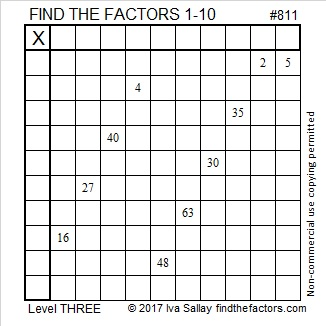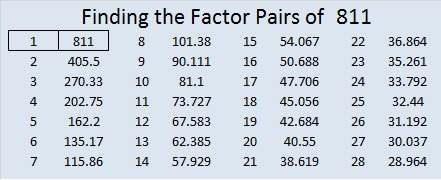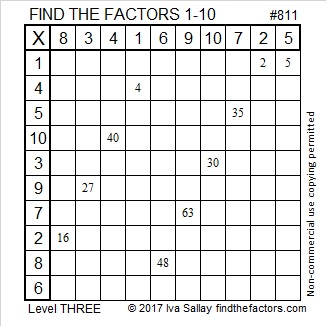# 811 My Youngest Grandchild

My youngest grandchild is 7 months old and is just beginning to crawl. I love the way he wipes drool off his own face in this video. It’s pretty funny.

Here’s today’s puzzle:Print the puzzles or type the solution on this excel file: 10-factors 807-814

• 811 is a prime number.
• Prime factorization: 811 is prime.
• The exponent of prime number 811 is 1. Adding 1 to that exponent we get (1 + 1) = 2. Therefore 811 has exactly 2 factors.
• Factors of 811: 1, 811
• Factor pairs: 811 = 1 x 811
• 811 has no square factors that allow its square root to be simplified. √811 ≈ 28.4780617How do we know that 811 is a prime number? If 811 were not a prime number, then it would be divisible by at least one prime number less than or equal to √811 ≈ 28.5. Since 811 cannot be divided evenly by 2, 3, 5, 7, 11, 13, 17, 19, or 23, we know that 811 is a prime number.

811 is the second half of a twin prime, and it is also the sum of five consecutive prime numbers:

• 151 + 157 + 163 + 167 + 173 = 811.

Stetson.edu informs us that the smallest prime factor of 24!+1 is 811. Wow! Really?

• If you type 24!+1 into your computer calculator you get 620,448,401,733,239,439,360,001.
• If you divide that number by 811, you get 765,041,185,860,961,084,291.
• If you type that into Number Empire’s Prime Number Checker, you will see that it is prime.• Thus 811 is the smaller of 24!+1’s two prime factors!1.Paula Beardell Krieg
•ivasallay